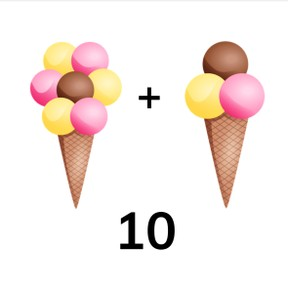# Adding to the number 10

I can add to the number 10.8,000 schools use Gynzy92,000 teachers use Gynzy1,600,000 students use Gynzy

## General

Students learn to add to the number 10. They know which two numbers together add to 10.

K.OA.A.2

## Relevance

Discuss with students that it is useful to be able to add, so you know how many of something you have. An example is if you eat an ice cream cone with 3 scoops, and your father has an ice cream cone with 7 scoops. You know that together you will eat 10 scoops of ice cream.

## Introduction

Ask students to form pairs. Give them 10 pencils and tell students that one student must set down a number of pencils, and the second student must compose 10. Take turns. After this, discuss the number bonds of 10. First show the number bonds with red and white beads, then with lambs, and finally it is not shown and students have to do it in their heads.

## Development

Discuss with students that there are different addition problems whose total is 10, and show this on the interactive whiteboard with a flower with 10 leaves. The flower keeps changing slightly. First you show a flower with 10 leaves of the same color. Next, you see a flower with 1 green leaf, and 9 purple leaves. This continues showing all the different pairs that make ten. Make sure to mention the addition problem that each flower represents. The flower always has 10 leaves, so the total of the addition problem is always 10.
Next show a squirrel with some acorns. Say that you can add the acorns by first counting the first group, and then counting on with the next group. Count the acorns out loud. Do not stop and count the second group separately, but count on from 4. Practice then with two examples using baby chicks and flowers, whose totals are also 10.
Explain that you might also see these problems without images, but only with numbers. You do the same, count the second number with the first number. Students can use blocks as support and set out as many blocks are in the first number and then use blocks to count on. As a class, discuss two addition problems that only show numbers whose total is 10.
Next show the squirrels with a few acorns. This time they don't need to add them, because they know the answer is 10, and they know how many acorns one squirrel has. You want to find out how many acorns the second squirrel has. Tell students that they can take the first number (which they have) and count on to 10. The number that they count is the number of acorns that the second squirrel has. Practice this again with the lambs and sheep, having students count the amount they have, and then counting how many they need to reach 10 to find the answer of how many the other animal has. Tell students that you can also find these problems without images, but only with numbers. You only see the first number, and then the answer which is 10. As a class, practice two exercises in which only numbers are visible.

Check that students understand adding to 10 by asking the following questions:
- How do you add two numbers together?
- What do you do if you want to figure out what the missing number is in the problem 4 + ... = 10?

## Guided practice

Students first practice adding with visual support. Next they must determine which numbers together add to 10.

## Closing

Discuss with students that it is important to be able to add to 10, because you can then do math problems and are able to determine how much you have of something. Ask the students to play a game of number card memory. They take turns turning over two number cards. If the two cards add to 10, they make take another turn. If they do not, the other player has a turn.

## Teaching tips

Students who have difficulty with addition can make use of MAB blocks or other manipulatives. Ask them to first count out the number they already have, and then to count on with more blocks to reach 10.

## Instruction materials

Number cards to 10.

### The online teaching platform for interactive whiteboards and displays in schools

• Save time building lessons

• Manage the classroom more efficiently

• Increase student engagement